K 66 M - Tuff Torq Transmission (2004-01)

Transmission

# K 66 M - Tuff Torq Transmission (2004-01) > Parts Diagrams (15) Hide 

•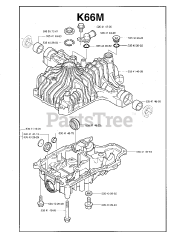Model K66M-A

•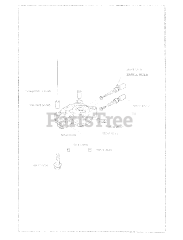Model K66M-B

•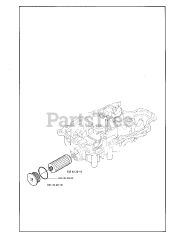Model K66M-C

•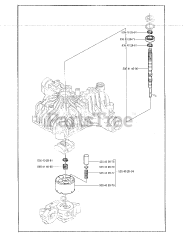Model K66M-D

•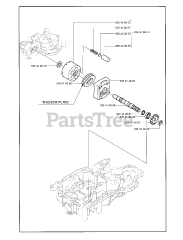Model K66M-E

•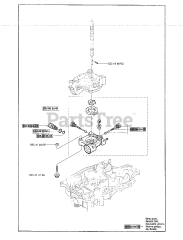Model K66M-F

•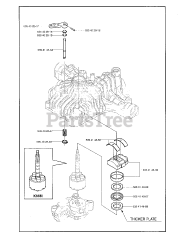Model K66M-G

•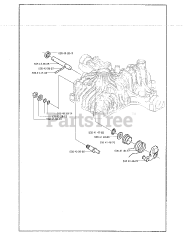Model K66M-H

•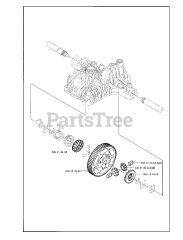Model K66M-J

•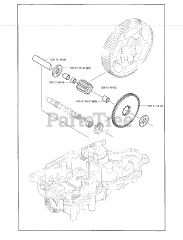Model K66M-K

•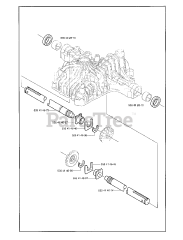Model K66M-L

•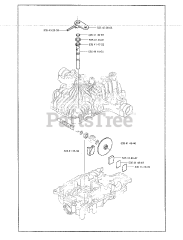Model K66M-M

•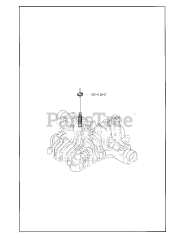Model K66M-N

•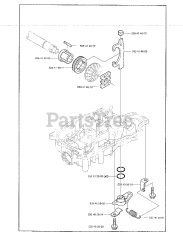Model K66M-P

•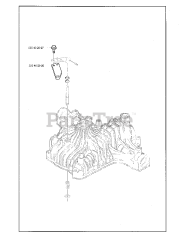Model K66M-Q